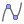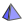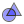## Free offline GeoGebra apps for iOS, Android, Windows, Mac, Chromebook and Linux

• ### Calculator Suite

Explore functions, solve equations, construct geometric shapes and 3D objects.

•### Graphing Calculator

Graph functions, investigate equations, and plot data with our free graphing app

•### 3D Calculator

Graph 3D functions, plot surfaces and do 3D geometry with our free 3D Grapher

•### Geometry

Construct circles, angles, transformations and more with our free geometry tool

• ### GeoGebra Classic 6

Apps bundle including free tools for geometry, spreadsheet, probability, and CAS

• ### CAS Calculator

Solve equations, expand and factor expressions, find derivatives and integrals

• ### GeoGebra Classic 5

Apps bundle including free tools for geometry, spreadsheet, probability, and CAS